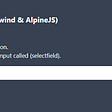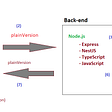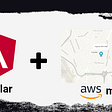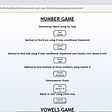# Scenario

We wish to have a function where we can pass in any number of arguments and add them together. So for example:

`sum(1,2)`

or

`sum(1,2,3)`

Also we want another function where we can subtract the first argument from the sum of the rest. Like this:

`addThenSubtract(1,2,3) // Result would be 4addThenSubtract(1,2,3,4) // Result would be 8`

The SPREAD operator is also expressed as `...` . We will see this used when we implement a`ddThenSubtract` .

# Approach

## Sum

We `implement` sum like this.

`function sum(...arr: number[]): number {        let sum = 0;        arr.forEach((v) => {        sum += v;    });    return sum;}`

The REST `…` operator collects all the arguments into an an array `arr` for us, and we then calculate the sum.

Here we can subtract the first argument from the sum of the rest.

`/** * @param subtract Subract this number from the numbers added * @param add Add tese numbers together */function addThenSubtract(subtract: number, ...add: number[]) {    console.log(`subtract \${subtract}`);    console.log(`add \${add}`);    const total = sum(...add);    return total - subtract;}`

Note that when we call `add` we use an operator that looks like the REST operator `...` . In this context it’s a SPREAD operator. It takes the values in the array and spreads them out. So if we pass in `[1,2,3]` it will become `1,2,3` .

# Demo

--

--

--

## More from Ole Ersoy

Founder of Firefly Semantics Corporation

Love podcasts or audiobooks? Learn on the go with our new app.

## Declarative GraphQL: write less code and get more done with graphql-tools## Unit testing State/Prop changes in React using react-test-renderer## Discord Bot on your voice channel## Writing custom virtual scroll strategy## Select Field with Search (Tailwind & AlpineJs)## Creating a Reactive Todo Application With the Firefly Semantics Slice State Manager## Nike Air Zoom Streak 5 https://t.co/7QcdQ8UYnn https://t.co/6lP1aBZyCR## Ole Ersoy

Founder of Firefly Semantics Corporation

## Easy alternatives for Map and Set in a JavaScript-oriented front-end, back-end configuration## Adding the map component using the AWS services to an Angular application## My Journey with DLithe Bootcamp for .NET full stack Developer Week2(MAR 27-APR02)## Classes in TypeScript